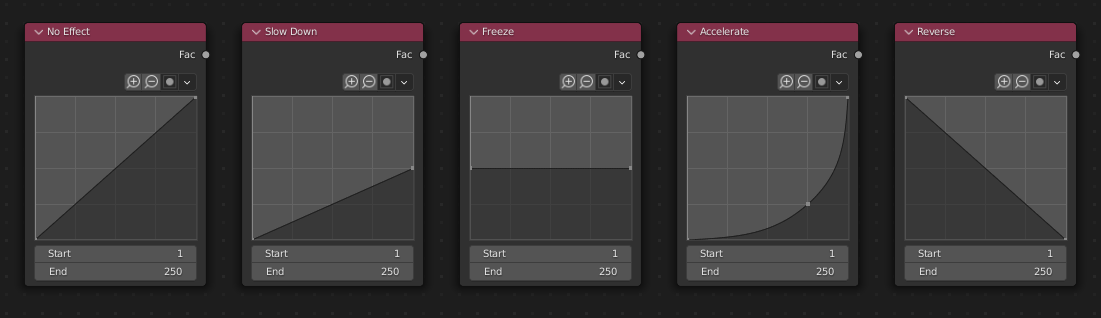# Time Curve Node

The Time Curve node generates a factor value (from 0.0 to 1.0) that changes according to the curve as time progresses through the Timeline.

## Inputs

This node has no inputs.

## Eigenschaften

Curve

The Y value defined by the curve is the factor output. For the curve controls see: Curve widget.

Tipp

Flipping the curve around reverses the time input, but doing so is easily overlooked in the node setup.

Start, End

Start frame and End frame of the range of time specifying the values the output should last. This range becomes the X axis of the graph. The time input could be reversed by specifying a start frame greater than the end frame.

## Outputs

Factor

A speed of time factor (from 0.0 to 1.0) relative to the scene’s Frame Rate. The factor changes according to the defined curve.

Hinweis

Output values

The Map Value node can be used to map the output to a more appropriate value. With sometimes curves, it is possible that the Time Curve node may output a number larger than one or less than zero. To be safe, use the Min/Max clamping function of the Map Value node to limit output.

## ExampleTime controls from left to right: no effect, slow down, freeze, accelerate, reverse.